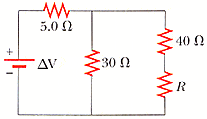Hi, I am having a bit of trouble solving for an unknown current. Can anyone help me out, thanks.

The resistor R in Fig18.56 dissipates 11 W of power. Determine 2 possible values of R (V= 76 V).My attempt so far,

1st loop: 35I-35I1=76

2nd loop: I=[(70/30)+(R/30)]I1

I plugged equation (2) into (1): 81.667I1 +1.1667I1R-35I1
=76...(3)
Given that:
I1R =11W/I1

plug that into (3): 46.667I1 +12.8337/I1 -76=0

I've tried using quadratic equation to solve for I1, then plugging into P=I^2R to solve for R, but I still keep getting the wrong answer. Please help. Thank you.

AlephZero
Homework Helper
1st loop: 35I-35I1=76

Are you sure about that? Why do you think both currents flowing through 35 ohms resistance?

Is it 35I-30I1=76? plug (2 )into that then solve with IR. In the end, do I use the quadratic equation to solve for I1? thanks.

AlephZero
Homework Helper
Is it 35I-30I1=76?/
That looks better.The rest of your first attempt was the right idea.

I attempted the problem again with the new 1st loop equation, however I am still getting the incorrect answer for I1 and R. Can someone help me solve the problem? Thanks.

yea, it's not 35I-30I1. I is supposed to be the current before the currents splits, right? im guessing you got 35 by adding 30 and 5 together, but when does the current I ever go across the 30 ohm resistor? by the time it reaches the 30 ohm resistor, the current is different cause it split.

also, im not sure how you got the equation for the second loop, neither I = I2 +I3... or V-RI-RI2-RI3.... would result in that.

AlephZero
Homework Helper
The OP was using I for the loop current in the first loop and I2 for the loop current in the second loop I think.

Using that notation my loop equations are

5 I + 30(I-I2) = 76
30(I-I2) = (40+R)I2

Eliminating I gives

1550 I2 = 2280 - 35 R I2

Power = R I2^2 = 11

So multiplying the equation by I2,

1550 I2^2 = 2280 I2 - 385

R = 6.76 or 20.36